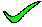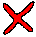There are no repeating terms, so at the very least the relation is in 1NF.

closeIf it was not in 2NF, then h,i or j would have to have a function dependency (either all together or some subset) which relates from a subset of e,f,g. Here, f,g->j, and so this cannot be in 2NF. It therefore follows it cannot be in 3NF or BCNF, so it must be in either 0NF or 1NF.

close3NF involves partial key dependencies. In this case, to NOT be in 3NF, e,f,g would have to be involved in selecting a subset of h,i,j. There are dependencies which produce this selection ( f,g => j ). Thus this relation IS NOT in 3NF, and thus cannot be in 4NF or BCNF.

close3NF involves partial key dependencies. In this case, to NOT be in 3NF, e,f,g would have to be involved in selecting a subset of h,i,j. There are dependencies which produce this selection ( f,g => j ). Thus this relation IS NOT in 3NF, and thus cannot be in 4NF or BCNF.

close3NF involves partial key dependencies. In this case, to NOT be in 3NF, e,f,g would have to be involved in selecting a subset of h,i,j. There are dependencies which produce this selection ( f,g => j ). Thus this relation IS NOT in 3NF, and thus cannot be in 4NF or BCNF.

close

 A B C D E TELL ME NEXT INDEX

Consider the following functional dependencies

 a,b => c,d e,g,h => f,j a,c => b,d p,q => r,s e,f,g => h,i s => t f,g => j q => u g,h => i

Which of the following best describes the relation R(e,f,g,h,i,j)?
1. First Normal Form
2. Second Normal Form
3. Third Normal Form
4. Forth Normal Form
5. Boyce Codd Normal Form